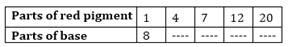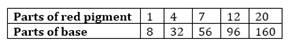# A mixture of paint is prepared by mixing 1 part of red pigments with 8 parts of base. In the following table, find the parts of base that need to be added.Solution

Let the ratio of parts of red pigment and parts of the base be a/b.

As the red pigment is in direct proportion with parts of the base,

i) 1/8 = 4/a1

So, a1 = 32

ii) 1/8 = 7/a2

So, a2 = 56

iii) 1/8 = 12/a3

So, a3 = 96

iv) 1/8 = 20/a4

So, a4 = 160

Hence, the required parts of the bases are